# Ratio Analysis Formula: How to Do the Analysis CorrectlyNow, actually what is the purpose of ratio analysis? Ratios are extremely important tools in financial analysis since they help financial analysts implement plans that improve interest coverage, leverage, reordering, financial structure, liquidity, and profitability. In other words, financial ratios help measure the relative performance of various financial facets that characterize a company’s financial health. Ratio analysis is the process of taking accounting and other financial data from a company’s financial statement and organize them in a way that helps you recognize the strengths and weaknesses of a company. We show you how to do ratio analysis correctly, which ratio analysis formula to use, as well as the weakness of ratio analysis. Continue reading for more useful info by our professional Excel assignment help.Image credit: Shutterstock

A large number of types of ratio analysis can be formulated to add meaning to accounting and financial data of an organization. But, while the use of these ratios can be useful, it can also lead to an information overload which can be cumbersome for the financial analysts. For this reason, the ratios can be grouped into five distinctive groupings covering the basic financial areas of a company that needs ratio financial analysis. They include; Profitability Ratios, Liquidity Ratios, Leverage Ratios, Valuation Ratios, and Management Efficiency Ratios.

### Profitability ratios

A profitability ratio is a measure of profitability, which is a means of measuring a business’s performance. In analyzing a company’s profitability ratios, several financial metrics are used to assess the business’s ability to generate profit compared to its expenses and other relevant costs incurred during a specific period. Every company is most concerned with its profitability and can use profitability ratios to determine the bottom line and its return to the investors. Profitability ratios can be classified into the following categories:

• Return on Equity (ROE): ROE measures how much a firm makes for each dollar an investor (owner) puts into it. This is ratio concerns the company’s equity holders the most since it measures their ability to make a profit on their equity investment. This is calculated by taking the amount of net income earned (NI) by the total equity of the shareholder—if you want to get the value in percentage, you can multiply the resulting quotient by 100. The higher the value, the better the company is doing using the investor’s money.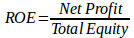• Return on Assets (ROA): ROA measures the amount of profit generated by each dollar of the company’s assets. In other words, this ratio measures how well (or effectively) the company generates its income from its assets. This is calculated by taking the company’s net income and dividing it by the value of the company’s total assets.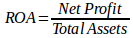• Net Profit Margin (NPM): NPM is the ratio of net profits to the company’s revenues. It is the commonly used profitability ratio as it compares the company’s bottom line to the amount of sales. NPM shows how each dollar accumulated by the firm as revenue translates into profit. It is calculated by taking the net profit by the total sales.• Gross Profit Margin (GPM): GPM is sometimes referred to as operating profit margin, and is the amount remaining after paying the cost of the sold goods (COGS). It essentially evaluates how much gross profit is generated from sales. Gross profit is equal to gross profit derived from net sales.

### Liquidity ratios

A business’s liquidity is its ability id its ability to meet its short-term financial obligations. Liquidity ratios measure a firm’s ability to pay off its current liabilities as they become due, and its long-term liabilities as they become current. Current liabilities are measured in relation to liquid assets to assess the coverage of immediate debts in cases of emergencies. The ratios discussed under Liquidity management include:

• Current Ratio (CR): CR is a liquidity ratio that measures the company’s ability to pay off its short-term liabilities with its current assets. Below is a formula used in calculating CR.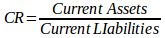• Quick Ratio (QR): QR (also referred to as Acid Test Ratio) is a liquid ratio that measures the firm’s ability to pay its current liabilities when they become due with only quick assets. Quick assets are those company assets that can be cashed in the short term (normally within 90 days). QR is calculated by dividing liquid assets by current liabilities.• Cash Ratio: Cash Ratio is the ratio of a business’s cash, as well as cash equivalent assets to its total liabilities, that can be used to pay its short-term obligations. A higher cash ratio generally means the firm is in a better position.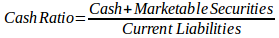• Working Capital (WC): WC is the amount of current assets in the company minus the current liabilities. It determines whether the organization can meet its current obligations with its current assets; as well as how much deficiency or excess there is.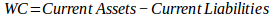### Leverage ratios

Leverage ratios measure the relative amount of funds supplied by equity and debt holders. Typically, businesses depend on a mixture of the owners’ equity and debt to fund their operations. A leverage ratio measures how much capital comes in the form of loans (debt) as well as assess if the company can meet its financial obligations. There are four types of leverage ratios including;

• Equity Ratio: This leverage ratio determines the portion of the total assets provided by the equity. Equity Ratios are calculated by dividing total equity by total assets. Equity Ratio can also be calculated by the formula—1 minus Debt Ratio. The reciprocal of equity ratio is called Equity Multiplier.• Debt Ratio: Debt Ratio measures the portion of the firm’s assets that is financed by the debt. It is calculated by dividing total liabilities by total assets. It can also be computed using the formula—1 minus Equity Rat.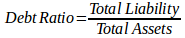• Debt-Equity Ratio: This evaluates the capital structure of the organization. A D/E (Debt/Equity) ratio that is more than 1 implies the business is a leveraged company. If the ratio is less than one, it implies the firm is a conservative one. Debt-Equity Ratio is calculated by dividing total liabilities by total equity.

###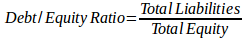Valuation ratios

Valuation Ratios put insight into the context of a firm’s share price where they serve as important tools for evaluating investment potential. There is a lot of Valuation Ratios, the following are some of the most important ones;

• Price-to-Earnings Ratio: Price-to-Earnings Ratio (P/E) looks at the relationship between the firm’s stock price and its earnings. Essentially, it is used to assess whether a stock is overpriced or underpriced. Investors expect a higher growth rate from businesses that have a higher P/E.• Dividend Yield Ratio: Dividend Yield Ratio measures the percentage of return through dividends against the price paid in for stock. You calculate this by dividing dividend per share by market price per share.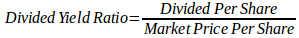• Price-to-Book Value Ratio: Price-to-Book Value Ratio (P/B) is the measures the value the market places on the book value of the company. The value book of a firm is the difference between the balance sheet liabilities and balance sheet assets. It is calculated by dividing current price per share by the book value per share.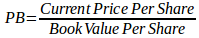• Earnings Per Share (EPS) Ratios: EPS ratio shows the rate of earnings per share of common stock. To calculate this ration, just deduct the preferred dividends from the net income and divide the result by the average common shares outstanding.### Management efficiency ratios

Management Efficiency Ratios, sometimes referred to as Asset Management Ratios or Efficiency Ratios, are ratios that measures the efficiency with which the firm manages its assets. There are several efficiency ratios in the long list of financial rations including:

• Accounts Payable Turnover (APT): Accounts Payable Turnover represents the number of times a firm can pay its accounts during a given period of time. It is calculated by taking net credit purchases and dividing by average accounts payable.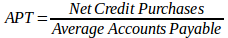• Inventory Turnover: The inventory turnover represents the number of times the inventory is sold and replaced. A bigger ratio means the firm is doing well in managing its inventories. It’s calculated by dividing the cost of sales by average inventory.• Days Inventory Outstanding (DIO): DIO represents the number of days the inventory sits in the warehouse. The shorter the DIO the better.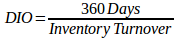• Days Sales Outstanding (DSO): Days Sales Outstanding is also known as “Collection Period” or “Receivable Turnover in Days” and it measures the average number of days a business takes to collect a receivable. It is calculated by dividing 360 days by the receivable turnover.• Receivable Turnover: Receivable Turnover measures the efficiency of extending and collecting credit. A higher ratio shows efficient credit and collection process. It is calculated by dividing net credit sales by average accounts receivable.• Total Asset Turnover (TAT): TAT measures the overall efficiency of a company in generating sales using its assets. You can calculate TAT by taking net sales and divide it by average total assets.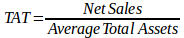As you can see, there are plenty of financial ratios, and each requires a specific formula to calculate. One of the areas where students do mistakes is identifying the information that has meaning for a specific use without becoming lost in inappropriate and unrelated ratios and data. Besides, the subject of ratio analysis is supplied with untested claims about which ratios should be used and what their suitable levels should be.

This has been a problem for many students over the years who have struggled to master the art of working out problems associated ratio analysis. This is where we come in! As the name suggests, Finance Homework Help helps you with finance assignments. Therefore, for this part, we show you how profitability, liquidity, efficiency, solvency, and all other performance aspects of a company can be calculated using ratio analysis.

It takes very little time to place an order at low cost with us, and financial experts will be ready to help you with ratio analysis problems, excel assignment help, limitations of ratio analysis, bond valuation formula, and profit margin problems that you have. We offer special discounts for finance assignment, and we have a live 24/7 chat tool to attend to your queries. You can also give us a call, and we’ll deliver your order on time.